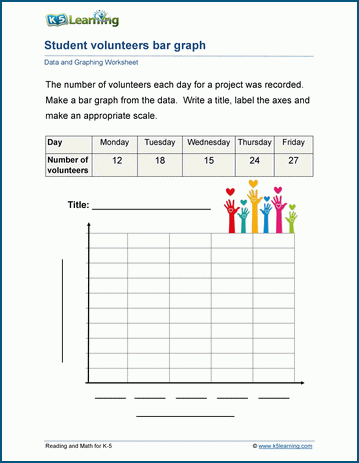# Data and Graphing Worksheets for Grade 3

The grade 3 data and graphing worksheets build on the skills learned in grades 1 and 2. Students now work on more complex data sets and are introduced to scaled graphs. Let us show you the new worksheets in this section.

## Graphing tally counts

Working from tally counts, students are asked to create bar graphs and line graphs in these worksheets.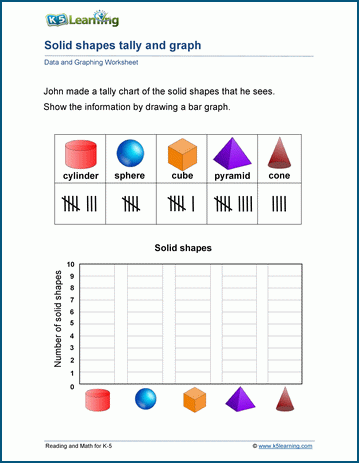## Spin, tally, graph and analyze worksheets

Students spin a pencil to create data, they tally each spin, then create bar graphs and analyze the data.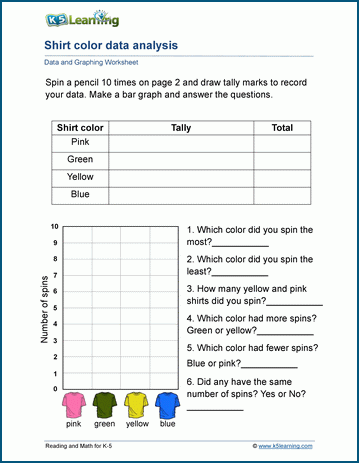## Scaled pictographs worksheets

Students work with pictographs to analyze the data in these worksheets.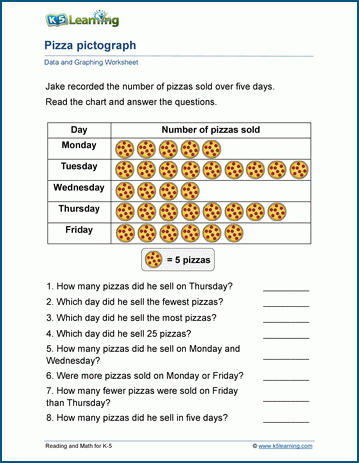## Scaled bar graphs

In these worksheets, students are given data and asked to draw bar graphs.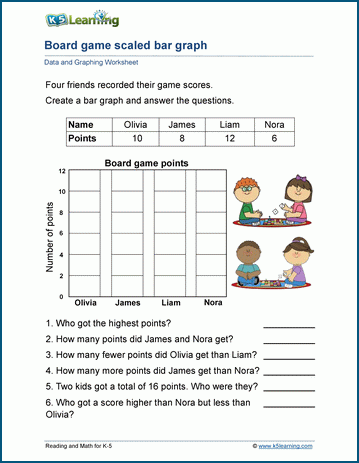## Students analyze scaled bar graphs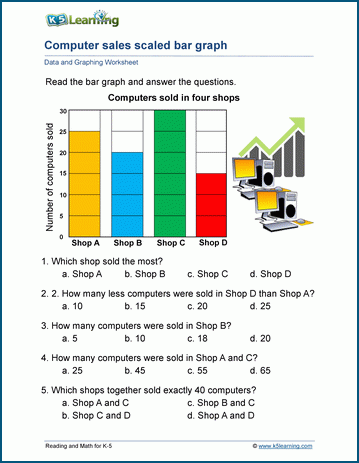## Worksheets on interpreting line plots

Similarly, students read and interpret line plots without the original data, in these worksheets.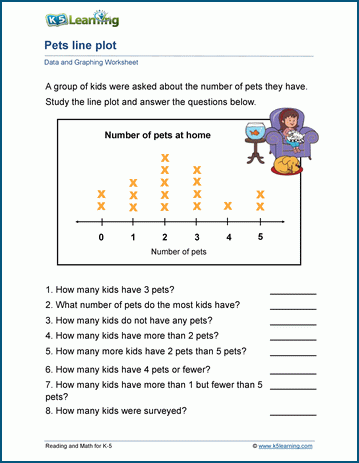## Students make line plots

Now students are asked to create line plots from data sets.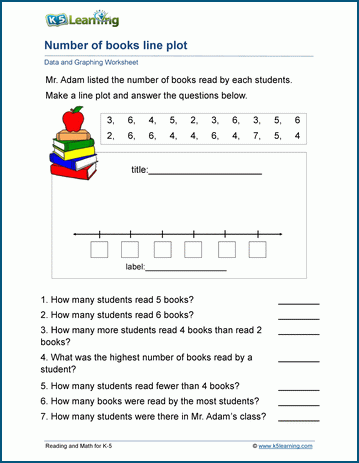## Circle graphs practice

The circle graph is a new concept for students, introduced in grade 3.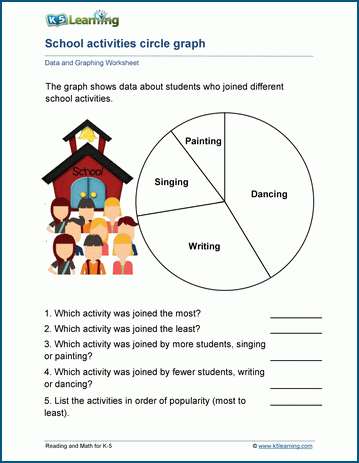## Students collect data

Students collect their own data and then draw bar graphs. We’ve provided a couple of example worksheets to get their creative juices flowing. It’s best to let students collect data on topics that interest them.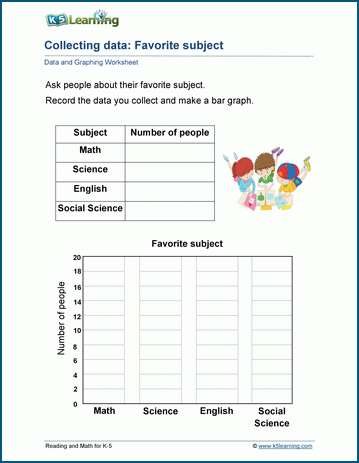## Double and triple Venn diagrams

Students practice mapping data sets into double and triple Venn diagrams in these worksheets.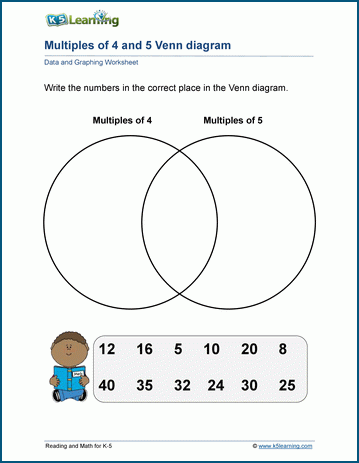## Line graphs work

These worksheets have students practicing line graphs.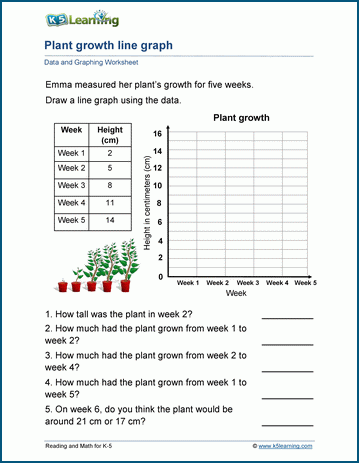## Labelling graphs worksheets

In these worksheets, students create graphs, including a title, a scale and labelling the axes for others to understand the data being analyzed.